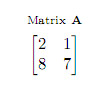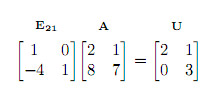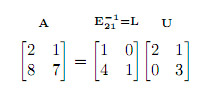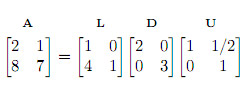This is the fourth post in an article series about MIT's Linear Algebra course. In this post I will review lecture four on factorizing a matrix A into a product of a lower-triangular matrix L and an upper-triangular matrix U, or in other words A=LU. The lecture also shows how to find the inverse of matrix product A·B, how to find the inverse of transposed matrix AT, and introduces permutation matrices.

Here are the previous lectures:

## Lecture 4: A=LU Factorization

Lecture four starts with a small review of inverse matrices. Remember from previous lecture that the inverse of matrix A is matrix A-1 such that multiplying them together A·A-1 produces the identity matrix I.

Another key fact to remember is that matrix multiplication is associative (can change parenthesis) and that for square matrices the right-inverse is also the left-inverse A·A-1 = A-1·A = I.

Lecture then continues with finding the inverse of matrix product A·B. The answer is found by reasoning what should we multiply A·B to get the identity matrix. Let's try B-1·A-1. If this is the inverse of A·B, then multiplying it with A·B from the left and right sides should produce the identity matrix I. Let's test this.

From the right side: (A·B)·(B-1·A-1). Since we can rearrange parenthesis, this is the same as A·(B·B-1)·A-1. But B·B-1 is identity, therefore A·(B·B-1)·A-1 = A·I·A-1 = A·A-1 = I. Seems good so far.

Now the left side: (B-1·A-1)·(A·B). We rearrange parenthesis again, B-1·(A-1·A)·B and just like in the previous example, A-1·A is identity and B-1·I·B is B-1·B = I.

We have found the inverse of (A·B). It's (B-1·A-1).

Next, while we're at it, the lecture continues with finding the inverse of transposed matrix AT. It's found by reasoning again. Let's first look at the equation A·A-1 = I. We can transpose both sides of this equation and it will still hold. If we transpose the right side, we get the same identity matrix I, because IT=I. But what about the left-hand side? Transposing the left-hand side we get (A·A-1)T = (A-1)T·AT. Now left-hand side is still equal to right-hand side, therefore (A-1)T·AT = I. But from this equation it's instantly obvious that the inverse of AT is (A-1)T.

We can therefore note and remember that (AT)-1 = (A-1)T.

Finally the lecture moves on to today's key topic of A=LU decomposition. As usual, the topic is introduced by an example.

Let's take a look at this 2x2 matrix A:And let's try to find the elementary matrix E21 that will eliminate the element at row 2, column 1. Multiplying the first row by 4 and subtracting it from the second row will produce a zero at 2, 1.But look at the right-hand side. It's the upper-triangular matrix U (all elements below the diagonal are 0) that we were looking for!

Now if we multiply both sides by the inverse of E21, we get (E21)-1·E21·A = (E21)-1·U. But (E21)-1·E21 is identity, therefore A = (E21)-1·U. From this equation it's instantly obvious that the lower-triangular matrix L is nothing else but (E21)-1!Another form of factorization is A = LDU, where D is the diagonal matrix that contains the pivots. For this example it would be:Now imagine that we have some arbitrary 3x3 matrix A. To reduce matrix A into upper-triangular matrix U, we first eliminate the element at position 2,1, that is, we multiply A (from the left side) with elimination matrix E21. Then we eliminate the element at 3,1 by multiplying (from the left side) with elimination matrix E31. Finally we eliminate the element at 3,2 by multiplying with elimination matrix E32:

E32·E31·E21·A = U

It follows from this equation that the lower-triangular matrix is the inverse of E32·E31·E21, that is,

L = (E32·E31·E21)-1 = E21-1·E31-1·E32-1

We have found the factorization of a 3x3 matrix:

A = E21-1·E31-1·E32-1·U = L·U

The algorithm for finding matrices L and U should now be clear. First do the elimination to find matrix U, then invert the product of elimination matrices used for finding U to find L. Actually it's even easier, you don't even need to keep elimination matrices E, or find their inverse. You can just keep the multipliers used in each elimination step. Please see the video to find out how it works.

Next, the cost of elimination algorithm is discussed. How many steps does it take to go from matrix A to U? Turns out the elimination is O(n3) process (more carefully, it takes around n3/3 steps). (See my notes on MIT's Introduction to Algorithms for more info about O-notation.)

Since we sometimes need to do row exchanged to do elimination, the last ten minutes of lecture are spent on permutation matrices. Remember from lecture two that multiplying a matrix from the left side with a permutation matrix exchanges its rows.

The key facts about permutation matrices P are:

• The inverse of P is its transpose: P-1 = PT.
• There are n! permutation matrices for nxn matrices.

Here is the video of the fourth lecture:

Topics covered in lecture four:

• [01:20] What is the inverse of A·B?
• [05:20] What is the inverse of AT?
• [09:20] A=LU factorization for 2x2 matrices.
• [13:20] A=LDU factorization.
• [14:50] A=LU decomposition for 3x3 matrices.
• [18:30] Why is finding matrix L trivial?
• [27:25] How many operations does elimination take?
• [42:20] Permutation matrices.
• [47:20] What is the inverse of a permutation matrix?
• [48:20] How many P matrices for 3x3 and 4x4 matrices?

Here are my notes of lecture four:

The next post is going to be about transpose matrices, symmetric matrices, vector spaces, their subspaces and column spaces of matrices.